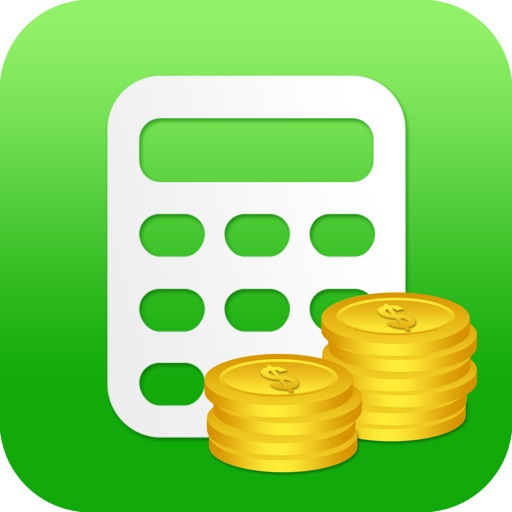#### Appgraphyサービス終了のお知らせ## EZ Financial Calculators Pro

• 価格：610JPY
•• バージョン：7.2.1
• 2013-03-15リリース

### アプリ詳細

For phone and tablet, this application includes the complete package of financial calculators by Bishinew Inc:

Finance and Investment Calculators

* TVM Calculator
* Currency Converter
* Compound Interest Calculator
* Return On Investment (ROI) Calculator
* IRR NPV Calculator
* MIRR Calculator
* Bond Calculator
* Tax Equivalent Yield Calculator
* Rule of 72 Calculator

Loan/Mortgage Calculators

* Loan Calculator
* Loan Comparison Calculator
* Loan Refinance Calculator
* APR Calculator
* Commercial Loan Calculator
* Simple Loan Calculator
* Loan Analysis Calculator
* Home Affordability Calculator
* Mortgage Tax Saving Calculator
* Discount Points Calculator
* Fixed vs Adjustable Rate Calculator
* Bi-weekly Payment Calculator
* Interest Only Calculator

Retirement Calculators

* Retirement Planner
* 401k Contribution Calculator
* Retirement Calculator
* Retirement Savings Analysis
* Retirement Income Analysis
* Traditional IRA vs Roth IRA
* Required Minimum Distribution
* Social Security Estimator
* Asset Allocation Calculator
* 401k Save the Max Calculator
* College Savings Calculator

Stock Calculators

* Stock Return Calculator
* Stock Constant Growth Calculator
* Stock Non-constant Growth Calculator
* CAPM Calculator
* Expected Return Calculator
* Holding Period Return Calculator
* Weighted Average Cost of Capital Calculator
* Pivot Point Calculator
* Fibonacci Calculator
* Black-Scholes Option Calculator

Credit Card Calculators

* Credit Card Payoff Calculator
* Credit Card Minimum Calculator

Auto Loan and Lease Calculators

* Auto Loan Calculator
* Auto Lease Calculator

Miscellaneous Calculators

* Regular Calculator
* Tip Calculator
* Discount and Tax Calculator
* Percentage Calculator
* Date Calculator
* Unit Conversion
* US Inflation Calculator
* Margin and Markup Calculator
* Fuel Calculator
* Salary Increase Calculator
* US Paycheck Tax Calculator
* Net Distribution Calculator
* Effective Rate Calculator
* Mutual Fund Fee Calculator
* Unit Price Compare Calculator
* Depreciation Calculator
* Break Even Point Calculator
* Financial Ratios

User can send the calculation results to others via Email. Financial Professionals can email the quote to their clients.

The app allows you to edit and prioritize the list of calculators for easy access.

Only Currency Converter needs internet access to retrieve the latest currency exchange rate. The app works without internet connection.

If you have any question or report issue, please send email to pfinanceapp@gmail.com. We will reply right away.

http://www.fncalculator.com

## 韓国のランキング推移